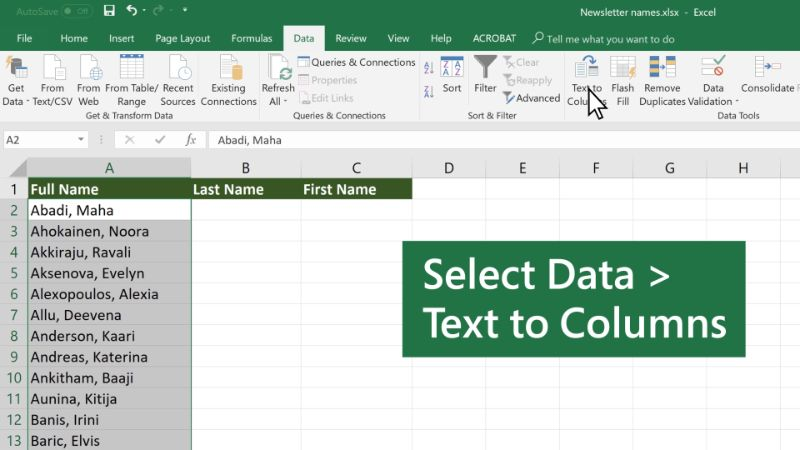# How to split a cell in Excel

With the following Excel tutorial, you can learn to divide a cell in Excel after having merged it with others. Among the many features that Excel incorporates, we find that of combining cells, this means that we have previously joined several Excel cells and converted them into a single cell. If after a while we want to have different cells again, we will have to learn to divide the cells in the spreadsheet. Today we are going to see how this process is done in order to present the information more adequately. And so we can use different ways to split a cell in Excel.

## The split a cell in Excel step by stepThis first Excel tutorial that we are going to see is how to divide an Excel cell once it is combined.

From 2 merged cells ( combined and centered ) we want to divide them, returning to their original situation. Once the cells have been combined in Excel  we can divide them by following the steps in the Excel tutorial :

We start from the combined and centered cells. We select the corresponding cell that we want to divide.

To divide a combined cell in Excel in two we will go to the ribbon to the home tab, look in the group of alignment options and select the arrow to the right of the combine and center button. (See image below)

Among all the options we have, we will select the option of Separate cells.

This will allow us to divide an Excel cell that has previously been merged.

## Split the contents of a cell into several cellsWe may have several types of data in an Excel cell and we want to divide the cell so that each of them has its own space. In order to divide the cell in this way, what we do is divide the contents of the cell and move it to several adjacent cells. The process is done automatically and it may seem that what we are actually doing is dividing the cell.

When is this Excel trick appropriate? When we open a CSV with the data separated by commas or periods and we want them to be separated into different cells. If you open an Excel file and discover that the contents of the cell are piled up you can separate it like this.

1. We select the range of cells we want to divide.
2. We go to the ribbon to the data tab.
3. Select Text in Columns.
4. A dialog box will appear where we will have to follow the steps that it indicates.

Step 1. We indicate that we want to delimit the data in columns.

Step 2. We indicate the way of separating said data to identify it and thus know where the cut mark is. In the case of the example (image below) they are period and like (;), but it is possible to indicate comma, space or another specific symbol.

• We indicate the format of the cell you will have, text, date, number …
• End operation

Easy right? Following these steps, we will convert the contents of a cell into several cells. Visibly it will appear that we have divided the Excel cell into several. This is one of the easy ways to split a cell in Excel.

### Ronney